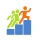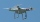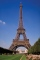# Angle + length - math problems

1. Observation towerFrom the observation tower at a height of 105 m above sea level, the ship is aimed at a depth angle of 1° 49´. How far is the ship from the base of the tower?
2. Length of the arcWhat is the length of the arc of a circle k (S, r=68mm), which belongs to a central angle of 78°?
3. What percentageWhat percentage of the Earth’s surface is seen by an astronaut from a height of h = 350 km. Take the Earth as a sphere with the radius R = 6370 km
4. StepsFind the height between the two floors if you know that the number of steps between the two floors is 18, the gradient is 30º and the length of the step is 28.6 cm. Report the result in centimeters to the nearest centimeter.
5. Triangular pyramidA regular tetrahedron is a triangular pyramid whose base and walls are identical equilateral triangles. Calculate the height of this body if the edge length is a = 8 cm
6. A droneA flying drone aimed the area for an architect. He took off perpendicularly from point C to point D. He was at a height of 300 m above the plane of ABC. The drone from point D pointed at a BDC angle of 43°. Calculate the distance between points C and B in
7. Three parallelsThe vertices of an equilateral triangle lie on 3 different parallel lines. The middle line is 5 m and 3 m distant from the end lines. Calculate the height of this triangle.
8. A rhombusA rhombus has sides of length 10 cm, and the angle between two adjacent sides is 76 degrees. Find the length of the longer diagonal of the rhombus.
9. The Eiffel TowerThe top of the Eiffel Tower is seen from a distance of 600 meters at an angle of 30 degrees. Find the tower height.
10. LighthouseMarcel (point J) lies in the grass and sees the top of the tent (point T) and behind it the top of the lighthouse (P). | TT '| = 1.2m, | PP '| = 36m, | JT '| = 5m. Marcel lies 15 meters away from the sea (M). Calculate the lighthouse distance from the sea
11. Cable carFind the elevation difference of the cable car when it rises by 67 per mille and the rope length is 930 m.
12. Prism diagonalThe body diagonal of a regular square prism has an angle of 60 degrees with the base, the edge length is 10 cm. What is the volume of the prism?
13. Balloon and bridgeFrom the balloon, which is 92 m above the bridge, one end of the bridge is seen at a depth angle of 37° and the second end at depth angle 30° 30 '. Calculate the length of the bridge.Mast has 13 m long shadow on a slope rising from the mast foot in the direction of the shadow angle at angle 15°. Determine the height of the mast, if the sun above the horizon is at angle 33°. Use the law of sines.
15. Depth angleFrom a cliff of 150 meters high, we can see the ship at a depth angle of 9° at sea. How far is the ship from the cliff?
16. Rectangular trapezoidThe ABCD rectangular trapezoid with the AB and CD bases is divided by the diagonal AC into two equilateral rectangular triangles. The length of the diagonal AC is 62cm. Calculate trapezium area in cm square and calculate how many differs perimeters of the
17. The mastThe top of the pole we see at an angle of 45°. If we approach the pole by 10 m, we see the top of the pole at an angle of 60°. What is the height of the pole?
18. Isosceles triangleThe circumference of the isosceles triangle is 32.5 dm. Base length is 153 cm. How long is the leg of this triangle?
19. DecagonCalculate the area and circumference of the regular decagon when its radius of a circle circumscribing is R = 1m
20. Area of iso-trapFind the area of an isosceles trapezoid if the lengths of its bases are 16 cm and 30 cm, and the diagonals are perpendicular to each other.

Do you have an interesting mathematical word problem that you can't solve it? Submit math problem, and we can try to solve it.

We will send a solution to your e-mail address. Solved examples are also published here. Please enter the e-mail correctly and check whether you don't have a full mailbox.

Please do not submit problems from current active competitions such as Mathematical Olympiad, correspondence seminars etc...

Do you want to convert length units?